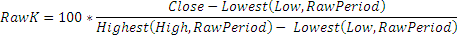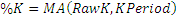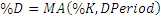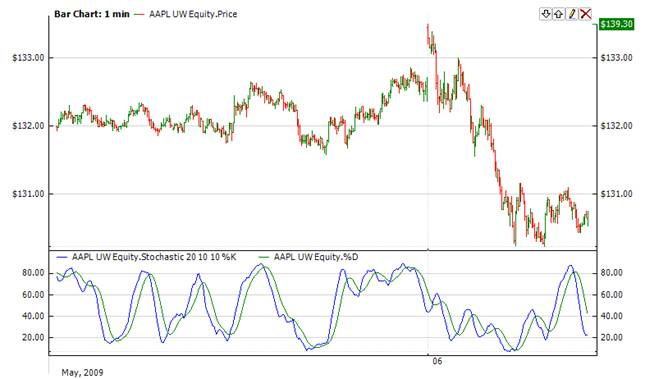﻿Stochastic Oscillator

# Stochastic Oscillator

The Stochastic compares where a security’s price closed relative to its price range over a given time period. The Stochastic is displayed as two lines. The main line is called %K. The second line, called %D, is a Moving Average of %K. The %K line is usually displayed as a solid line and the %D line is usually displayed as a dotted line.Market Signals

Buy when the Oscillator (either %K or %D) falls below a specific level (e.g., 20) and then rises above that level. Sell when the Oscillator rises above a specific level (e.g., 80) and then falls below that level.CalculationChart ExampleImplementation and Usage

To initialize Stochastic indicator use one of the following constructors:

Stochastic – sets default values: rawPeriod = 14, kPeriod = 3, dPeriod = 3

Stochastic(Int32, Int32, Int32) – sets values for periods

Stochastic(Int32, IAverager, IAverager) – sets raw periods and the type of averaging

Use

RawStochastic

PercentK

PercentD

properties to get current valueExample
C#
``` 1// Create new instance
2Stochastic stochastic = new Stochastic(28, 9, 6);
3
4// Number of stored values
5stochastic.HistoryCapacity = 2;
6
9
10// Get indicator value
11double IndicatorValue = stochastic.PercentK;
12double IndicatorValue = stochastic.PercentD;
13// Get previous value
14if (stochastic.HistoryCount == 2)
15{
16    double IndicatorPrevValue = stochastic.PercentK;
17    double IndicatorPrevValue = stochastic.PercentD;
18}```

Stochastic with custom averaging

C#
``` 1// Create new instance
2// Sets raw period = 28